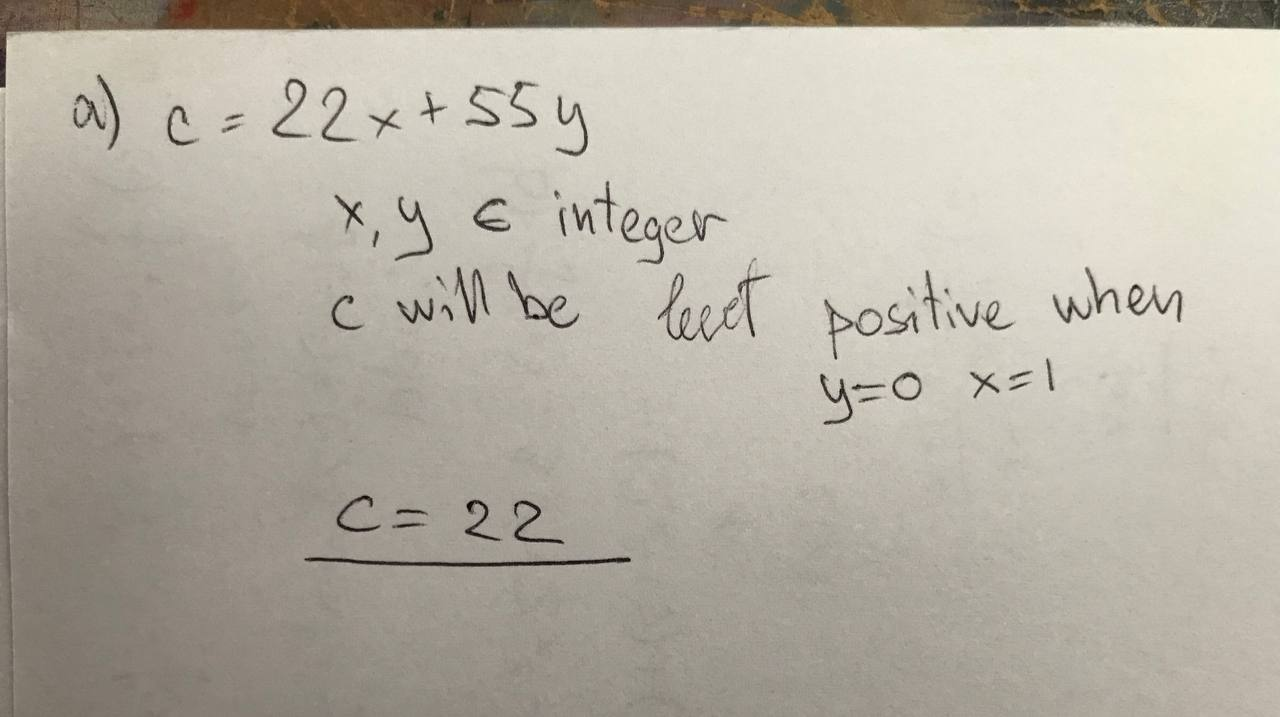# a. what is the least positive intergers c of the form c=22x+55y with x,y are integer. explain b. let c be the answer in part a. find integers x and y such that c=22x+55y and 0<x<5detineerlf 2022-08-01 Answered
a. what is the least positive intergers c of the form c=22x+55y with x,y are integer. explain
b. let c be the answer in part a. find integers x and y such that c=22x+55y and 0
You can still ask an expert for help

• Questions are typically answered in as fast as 30 minutes

Solve your problem for the price of one coffee

• Math expert for every subject
• Pay only if we can solve itEmilie Reeves###### Not exactly what you’re looking for?Urijah Estes